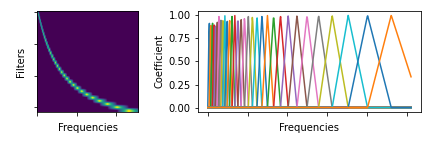Shortcuts

# torchaudio.prototype.functional¶

## barkscale_fbanks¶

torchaudio.prototype.functional.barkscale_fbanks(n_freqs: int, f_min: float, f_max: float, n_barks: int, sample_rate: int, bark_scale: str = 'traunmuller') [source]

Create a frequency bin conversion matrix.Parameters:
• n_freqs (int) – Number of frequencies to highlight/apply

• f_min (float) – Minimum frequency (Hz)

• f_max (float) – Maximum frequency (Hz)

• n_barks (int) – Number of mel filterbanks

• sample_rate (int) – Sample rate of the audio waveform

• bark_scale (str, optional) – Scale to use: traunmuller,schroeder or wang. (Default: traunmuller)

Returns:

Triangular filter banks (fb matrix) of size (n_freqs, n_barks) meaning number of frequencies to highlight/apply to x the number of filterbanks. Each column is a filterbank so that assuming there is a matrix A of size (…, n_freqs), the applied result would be A * barkscale_fbanks(A.size(-1), ...).

Return type:

torch.Tensor

## DSP¶

 adsr_envelope Generate ADSR Envelope filter_waveform Applies filters along time axis of the given waveform. extend_pitch Extend the given time series values with multipliers of them. oscillator_bank Synthesize waveform from the given instantaneous frequencies and amplitudes. sinc_impulse_response Create windowed-sinc impulse response for given cutoff frequencies. frequency_impulse_response Create filter from desired frequency response

## Room Impulse Response Simulation¶

 simulate_rir_ism Compute Room Impulse Response (RIR) based on the image source method .

## Docs

Access comprehensive developer documentation for PyTorch

View Docs

## Tutorials

Get in-depth tutorials for beginners and advanced developers

View Tutorials